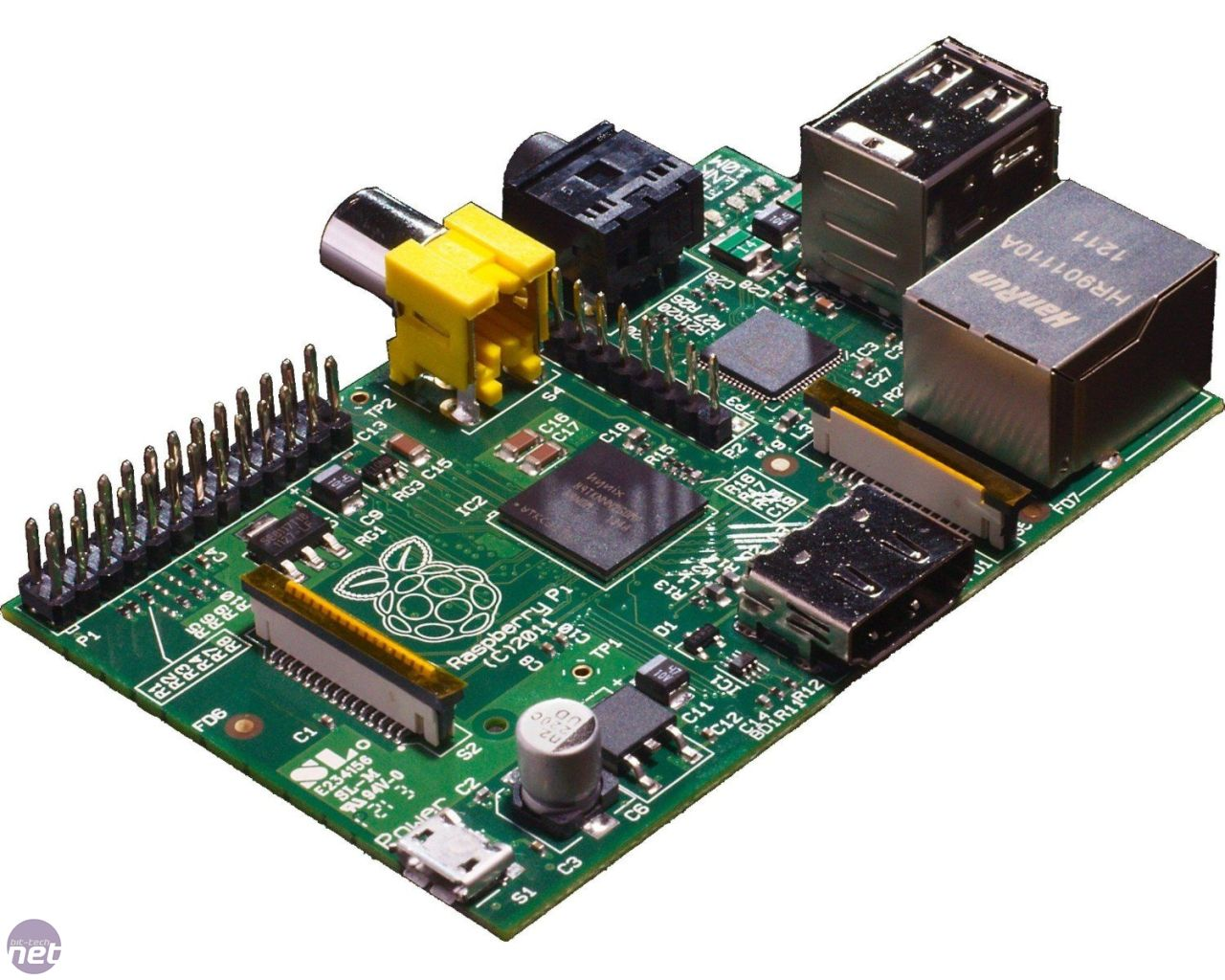## Graphics Waterfall

### Senior Project at Mahidol University International College

Inspiration: One of our professors, Graham K. Rogers, donated his raspberryPi to us, assuming we MIGHT want to work on an embedded system project.The question then becomes, ‘what are we going to do with it?’

Upon browsing around for projects, one of our juniors shared a youtube video with us:

The project starts with one raspberryPi and after three months our dreams become true.

Member:Advisors: (I) Dr. Arnan Sipitakiat (II) Asst. Prof. Thanadol Pritranan (III) Asst. Prof. Ittichote ChuckpaiwongStudent
Bottom:(I) Bhinyaporn Pattanasethanon (II) Kamonnate Cheevaprawatdomrong (III)Deeprom Somkiadchareon

In this project my tasks are:

– Code Image Pattern/ Text input functions in Python

– Assign and connect I/O ports to the software.

– Testing the electronic design of the embedded system.### Simple all off operation:

```import wiringpi2

pin_base = 65

wiringpi2.wiringPiSetup()

# reset all pins as outputs and turn off
for pin in range(65,100):
wiringpi2.pinMode(pin,1)
wiringpi2.digitalWrite(pin,0) # for all on {wiringpi2.digitalWrite(pin,1)}```

Code for Image inputs:

``` def _genimage(*img):

inputt= img

a=1*(a>0)

for i in range(0,len(a)):
pin=65
for j in range(0,32):
wiringpi2.digitalWrite(pin,int(a[i,j,1]))
pin=pin+1
wiringpi2.delay(65)

for pin in range(65,97):
wiringpi2.digitalWrite(pin,0)
wiringpi2.delay(800)```

Algorithm:

1. The general idea is to read the image and convert it to binary image.

2. Run a loop that assigns 32 pin ports to the right I/O instruction, row-wise.

3. After each row’s execution, assign a delay for 800 ms to turn off all ports.

4. Assign I/O ports to the next row.

Text Inputs:

There are two types of text inputs in this project.

Case input : A-B-C

Output shown:

A

B

C

Case input: abcd

Output shown:

AB

CD

[Because the resolution of this prototype is 32X8 pixels, we can only shrink 2 letters in one display to perform a droplet formation that human eye can distinguish. ]
``` def _gentext(*item):
# reset all pins as outputs and turn off
for pin in range(65,97):
wiringpi2.pinMode(pin,1)
wiringpi2.digitalWrite(pin,0)

inputt = item

#Case printing individual letters [-] inputs
if ('-' in inputt) | (len(inputt) ==1) :
texts = inputt.split('-')
for i in range(0,len(inputt)):
path='./TextfileWF/'+str.upper(inputt[i])
print(str.upper(inputt[i]))
x=file(path,'r+',255);
flip_a= flipud(a);
for i in range(0,len(a)):
pin=65;
for j in flip_a[i]:
if j!='\n':
wiringpi2.digitalWrite(pin,(int(j)))
pin+=1;
wiringpi2.delay(65)
for pin in range(65,97):
wiringpi2.digitalWrite(pin,0)
wiringpi2.delay(800)

# Case displaying 2 letters at the time

else:
texts = inputt.strip()
if len(texts)%2==0 :
for i in range(0,len(texts)):
if i%2==0:
w1='./TextfileWF/'+texts[i]
w2='./TextfileWF/'+texts[i+1]
with open('./TextfileWF/output','w') as g, open(w1) as word1, open(w2) as word2:
lis=[x1.split() for x1 in word1]
lis2=[x2.split() for x2 in word2]
for x1,x2 in izip(lis,lis2):
for y in x1:
for i in range(0,31):
if i%2==0:
g.write(y[i])
for n in x2:
for i in range(0,31):
if i%2==0:
g.write(n[i])
g.write('\n')

#Case the input word has odd amount of letter
else:
texts=texts+'*'
for i in range(0,len(texts)):
if i%2==0:
w1='./TextfileWF/'+texts[i]
w2='./TextfileWF/'+texts[i+1]
with open('./TextfileWF/output','w') as g, open(w1) as word1, open(w2) as word2:
lis=[x1.split() for x1 in word1]
lis2=[x2.split() for x2 in word2]
for x1,x2 in izip(lis,lis2):
for y in x1:
for i in range(0,31):
if i%2==0:
g.write(y[i])
for n in x2:
for i in range(0,31):
if i%2==0:
g.write(n[i])
g.write('\n')
for i in range(0,len(inputt)):
path='./TextfileWF/output'
x=file(path,'r+',255);
flip_a= flipud(a);
for i in range(0,len(a)):
pin=65;
for j in flip_a[i]:
if j!='\n':
wiringpi2.digitalWrite(pin,(int(j)))
pin+=1;
wiringpi2.delay(65)
for pin in range(65,97):
wiringpi2.digitalWrite(pin,0)
wiringpi2.delay(800)```

General Idea for the algorithm:

(case not sensitive)

1) Check if the input has [-] or not

Case input has dash: [A-B-C]

– split the [-]

-call text file

Case input is in word [ABCD|abdc] with even length

-strip text

– modulo text length by 2

-open both alphabet files

-scale down by 2

– write output into one output file

Case word input has odd length [ABC|abc]

– follow even length case

-add blank file to the last letter

Printing the output from file:

-flip the file upside down [to print from the bottom]

– assign pin number to I/O ports

From the Interface:To get the latest update of me and my works

>> <<